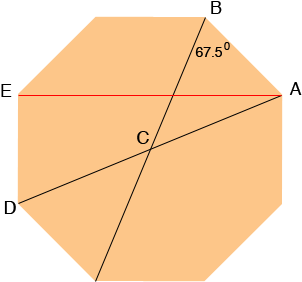SEARCH HOMEMath Central Quandaries & QueriesHi, Need to lay out a 10 ft. diameter octagon on the ground. What is the length of the sides ? And the angles ? Thanks , FrankHi Frank,I think the angle you need is the angle ABC which measures 67.5 degrees.

Suppose the side length AB is s feet. I'm not sure which distance is 10 feet.

If it is the distance from A to E then as Stephen showed s is 0.4142 times the distance from A to E so
s = 4.142 feet.

If the distance from A to D is 10 feet then since triangle AED is a right triangle we can use Pythagoras' theorem to find s. |ED| = 1/0.4142 s and |ED| = s so

|AD|2 = 1/0.41422 s2 + s2

and hence

102 = (3.4142)s2.

Thus

s2 = 100/3.4142

and

s = 5.41 feet

I hope this helps,
HarleyMath Central is supported by the University of Regina and The Pacific Institute for the Mathematical Sciences.